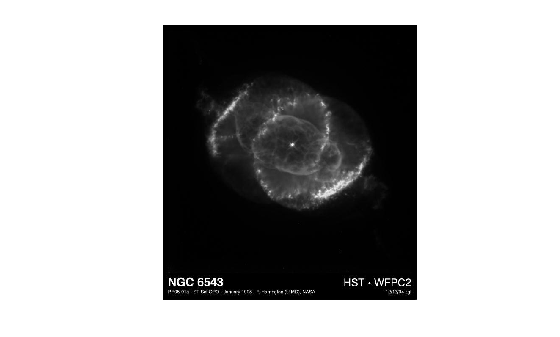# im2gray

Convert RGB image to grayscale

## Syntax

``I = im2gray(RGB)``

## Description

example

````I = im2gray(RGB)` converts the specified truecolor image `RGB` to a grayscale intensity image `I`. The `im2gray` function accepts grayscale images as inputs and returns them unmodified.The `im2gray` function converts RGB images to grayscale by eliminating the hue and saturation information while retaining the luminance.```

## Examples

collapse all

Read a truecolor (RGB) image into the workspace from a file and display it.

```RGB = imread('example.tif'); imshow(RGB)```Convert the RGB image into a grayscale image.

`I = im2gray(RGB);`

Display the converted grayscale image.

`imshow(I)`## Input Arguments

collapse all

Truecolor image, specified as an m-by-n-by-3 numeric array. `im2gray` also accepts m-by-n numeric arrays (grayscale images) and returns them unmodified.

If you have Parallel Computing Toolbox™ installed, `RGB` can also be a `gpuArray`.

Data Types: `single` | `double` | `uint8` | `uint16`

## Output Arguments

collapse all

Grayscale image, returned as an m-by-n numeric array. If the input to `im2gray` is a grayscale image, the output image `I` is the same as the input image.

If you have Parallel Computing Toolbox installed, then `I` can also be a gpuArray.

## Tips

• The `im2gray` function is identical to `rgb2gray` except that it can accept grayscale images as inputs, returning them unmodified. The `rgb2gray` function returns an error if the input image is a grayscale image. If you use the `im2gray` function, code like this loop is no longer necessary.

```if ndims(I) == 3 I = rgb2gray(I); end ```
• Unlike the `rgb2gray` function, the `im2gray` function does not accept colormaps as an input. To convert a colormap to grayscale, use the `cmap2gray` function.

## Algorithms

The `im2gray` function converts RGB values to grayscale values by forming a weighted sum of the R, G, and B components:

```0.2989 * R + 0.5870 * G + 0.1140 * B ```

These are the same weights used by the `rgb2ntsc` (Image Processing Toolbox) function to compute the Y component.

The coefficients used to calculate grayscale values in the `im2gray` function are identical to those used to calculate luminance (E'y) in Rec.ITU-R BT.601-7 after rounding to three decimal places.

Rec.ITU-R BT.601-7 calculates E'y using the following formula:

```0.299 * R + 0.587 * G + 0.114 * B ```

## Version History

Introduced in R2020b